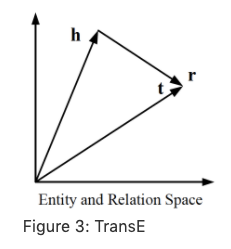# CompGCN

Multi-relational graphs are a more general and prevalent form of graphs where each edge has a label and direction associated with it. However, the primary focus has been on handling simple undirected graphs and the approaches tackling multi relational hetero-graphs often suffer from over-parameterization (like R-GCN) with most of them restricted to learning node representations only. Hence, such methods are not directly applicable for tasks such as link prediction which require relation embedding vectors. By definition, it’s clear that relations are extremely important in these hetero-graphs (eg. KGs). The goal is to learn representations in a relational graph of both the nodes and relations by jointly embedding them.

CompGCN introduced in this paper is a method leveraging a variety of entity-relation composition operations from Knowledge Graph Embedding techniques (TransE, TransR, RotatE, DistMul etc.) and scales with the number of relations.Composition-Based Multi-Relational Graph Convolutional Networks (CompGCN)

## KG Embeddings (KGEs)

A knowledge graph (KG) is a directed heterogeneous multigraph whose node and relation types have domain-specific semantics. Knowledge graph embeddings (KGEs) are low-dimensional representations of the entities and relations in a knowledge graph. They provide a generalizable context about the overall KG that can be used to infer relations. Most of KG embedding approaches define a score function and train node and relation embeddings such that valid triples are assigned a higher score than the invalid ones.Above figure illustrates an example of TransE method for KG Embedding. TransE is based on Additive score function. There are other methods which are based on Multiplicative or Neural scoring.

### GCN on Multi-Relational Graphs

For a multi-relational graph G = (V,R,E,X), where R denotes the set of relations, and each edge (u, v, r) represents that the relation r ∈ R exist from node u to v. The GCN formulation as devised by Marcheggiani & Titov (2017) is based on the assumption that information in a directed edge flows along both directions. Hence, for each edge (u, v, r) ∈ E, an inverse edge (v, u, r−1) is included in G. The representations obtained after k layers of directed GCN is given by

Here, denotes the relation specific parameters of the model. However, the above formulation leads to over-parameterization with an increase in the number of relations and hence, Marcheggiani & Titov (2017) use direction-specific weight matrices. Schlichtkrull et al. (2017) in R-GCN address over-parameterization by proposing basis and block-diagonal decomposition of Wrk.Activations (d-dimensional vectors) from neighboring nodes (dark blue) are gathered and then transformed for each relation type individually (for both in- and out- edges). The resulting representation (green) is accumulated in a (normalized) sum and passed through an activation function (such as the ReLU).

### CompGCN Formulation

Following Directed-GCN and Relational-GCN, CompGCN also allows the information in a directed edge to flow along both directions. Hence, E and R are extended with corresponding inverse edges and relations, i.e., and where denotes the inverse relations and T indicates the self loop.

Unlike most of the existing methods which embed only nodes in the graph, COMPGCN learns a d-dimensional representation hr along with node embeddings hv. Representing relations as vectors alleviates the problem of over-parameterization while applying GCNs on relational graphs. Further, it allows COMPGCN to exploit any available relation features (Z) as initial representations.

To incorporate relational embeddings into GCN, the composition operators from KG Embeddings are used.

where denotes initial features for node u and relation r respectively, hv denotes the updated representation of node v, and Wλ(r) is a relation-type specific parameter. In COMPGCN, we use direction specific weights, i.e., λ(r) = dir(r), given as:

Further, in CompGCN, after the node embedding update, the relation embeddings are also transformed as follows:

where Wrel is a learnable transformation matrix which projects all the relations to the same embedding space as nodes and allows them to be utilized in the next CompGCN layer.

## Key Contributions

• A novel Graph Convolutional based framework for multi-relational graphs which leverages a variety of composition operators from Knowledge Graph embedding techniques to jointly embed nodes and relations in a graph.

• Generalizes several existing multi-relational GCN methods.

• Alleviates the problem of over-parameterization by sharing relation embeddings across layers and using basis decomposition.

• The choice of composition operation is important in deciding the quality of the learned embeddings. Hence, superior composition operations for Knowledge Graphs developed in future can be adopted to improve COMPGCN’s performance further.